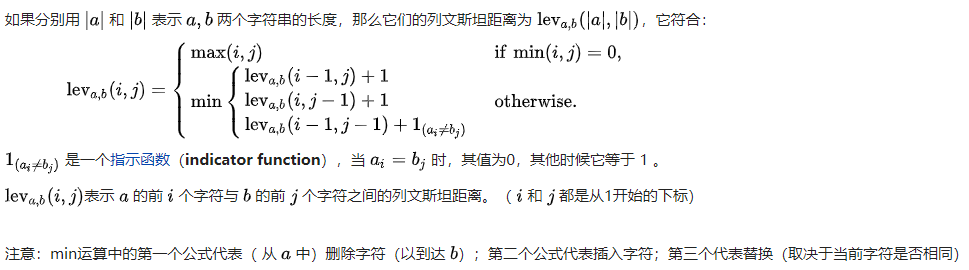# Levenshtein Distance（编辑距离）算法与使用场景

## 什么是Levenshtein Distance

Levenshtein Distance，一般称为编辑距离（Edit DistanceLevenshtein Distance只是编辑距离的其中一种）或者莱文斯坦距离，算法概念是俄罗斯科学家弗拉基米尔·莱文斯坦（Levenshtein · Vladimir I）在1965年提出。此算法的概念很简单：Levenshtein Distance两个字串之间，由一个转换成另一个所需的最少编辑操作次数，允许的编辑操作包括：

• 将其中一个字符替换成另一个字符（Substitutions）。
• 插入一个字符（Insertions）。
• 删除一个字符（Deletions）。

### Levenshtein Distance公式定义kitten这个单词转成sittingLD值为3：

1. kitten → sitten （k→s）
2. sitten → sittin （e→i）
3. sittin → sitting （insert a ‘g’）

### Levenshtein Distance动态规划方法

• 初始化一个LD矩阵(M,N)MN分别是两个输入字符串的长度。
• 矩阵可以从左上角到右下角进行填充，每个水平或垂直跳转分别对应于一个插入或一个删除。
• 通过定义每个操作的成本为1，如果两个字符串不匹配，则对角跳转的代价为1，否则为0，简单来说就是：
• 如果[i][j]位置的两个字符串相等，则从[i][j]位置左加1，上加1，左上加0，然后从这三个数中取出最小的值填充到[i][j]
• 如果[i][j]位置的两个字符串相等，则从[i][j]位置左、左上、上三个位置的值中取最小值，这个最小值加1（或者说这三个值都加1然后取最小值），然后填充到[i][j]
• 按照上面规则LD矩阵(M,N)填充完毕后，最终矩阵右下角的数字就是两个字符串的LD值。

• 例子一（两个等长字符串）：sonsun
• 例子二（两个非等长字符串）：dogedog

s o n
0 1 2 3
s 1
u 2
n 3

s o n
0 1 2 3
s 1 0
u 2
n 3

s o n
0 1 2 3
s 1 0 1 2
u 2 1 1 2
n 3 2 2 1

d o g
0 1 2 3
d 1
o 2
g 3
e 4

d o g
0 1 2 3
d 1 0 1 2
o 2 1 0 1
g 3 2 1 0
e 4 3 2 1

## Levenshtein Distance算法实现

public enum LevenshteinDistance {

// 单例
X;

/**
* 计算Levenshtein Distance
*/
public int ld(String source, String target) {
Optional.ofNullable(source).orElseThrow(() -> new IllegalArgumentException("source"));
Optional.ofNullable(target).orElseThrow(() -> new IllegalArgumentException("target"));
int sl = source.length();
int tl = target.length();
// 定义矩阵,行列都要加1
int[][] matrix = new int[sl + 1][tl + 1];
// 首行首列赋值
for (int k = 0; k <= sl; k++) {
matrix[k] = k;
}
for (int k = 0; k <= tl; k++) {
matrix[k] = k;
}
// 定义临时的编辑消耗
int cost;
for (int i = 1; i <= sl; i++) {
for (int j = 1; j <= tl; j++) {
if (source.charAt(i - 1) == target.charAt(j - 1)) {
cost = 0;
} else {
cost = 1;
}
matrix[i][j] = min(
// 左上
matrix[i - 1][j - 1] + cost,
// 右上
matrix[i][j - 1] + 1,
// 左边
matrix[i - 1][j] + 1
);
}
}
return matrix[sl][tl];
}

private int min(int x, int y, int z) {
return Math.min(x, Math.min(y, z));
}

/**
* 计算匹配度match rate
*/
public BigDecimal mr(String source, String target) {
int ld = ld(source, target);
// 1 - ld / max(len1,len2)
return BigDecimal.ONE.subtract(BigDecimal.valueOf(ld)
.divide(BigDecimal.valueOf(Math.max(source.length(), target.length())), 2, BigDecimal.ROUND_HALF_UP));
}
}


public static void main(String[] args) throws Exception {
String s = "doge";
String t = "dog";
System.out.println("Levenshtein Distance:" +LevenshteinDistance.X.ld(s, t));
System.out.println("Match Rate:" +LevenshteinDistance.X.mr(s, t));
}
// 输出
Levenshtein Distance:1
Match Rate:0.75


## Levenshtein Distance算法一些使用场景

LD算法主要的应用场景有：

• DNA分析。
• 拼写检查。
• 语音识别。
• 抄袭侦测。
• 等等…

### 脱敏数据和明文数据匹配

张*狗 123****8910 123456****8765****

张大狗 12345678910 123456789987654321

public static void main(String[] args) throws Exception {
String sourceName = "张*狗";
String sourcePhone = "123****8910";
String sourceIdentityNo = "123456****8765****";
String targetName = "张大狗";
String targetPhone = "12345678910";
String targetIdentityNo = "123456789987654321";
boolean match = LevenshteinDistance.X.ld(sourceName, targetName) == 1 &&
LevenshteinDistance.X.ld(sourcePhone, targetPhone) == 4 &&
LevenshteinDistance.X.ld(sourceIdentityNo, targetIdentityNo) == 8;
System.out.println("是否匹配:" + match);
targetName = "张大doge";
match = LevenshteinDistance.X.ld(sourceName, targetName) == 1 &&
LevenshteinDistance.X.ld(sourcePhone, targetPhone) == 4 &&
LevenshteinDistance.X.ld(sourceIdentityNo, targetIdentityNo) == 8;
System.out.println("是否匹配:" + match);
}
// 输出结果



### 拼写检查

public static void main(String[] args) throws Exception {
String target = "throwab";
// 模拟一个单词库
List<String> words = Lists.newArrayList();
Map<String, BigDecimal> result = Maps.newHashMap();
words.forEach(x -> result.put(x, LevenshteinDistance.X.mr(x, target)));
System.out.println("输入值为:" + target);
result.forEach((k, v) -> System.out.println(String.format("候选值:%s,匹配度:%s", k, v)));
}
// 输出结果



### 抄袭侦测

System.out.println(LevenshteinDistance.X.mr("我是一只小小小小鸟，想要飞呀飞却飞也飞不高", "我是一条小小小小狗，想要睡呀睡却睡也睡不够"));
// 输出如下
0.67